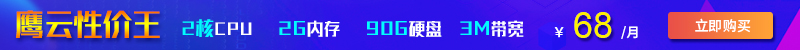php中二维数组求和用的函数有哪些这篇文章分享给大家的内容是关于php中二维数组求和用的函数有哪些，本文介绍得很详细，内容有一定的参考价值，能帮助大家进一步学习和理解“php中二维数组求和用的函数有哪些”，有这方面学习需要的朋友可以看看，接下来就让小编带领大家一起来学习一下吧。

php中二维数组求和只用array_sum()是不行的，因为array_sum()函数只处理一维数组；想要使用array_sum()实现二维数组求和，还需要借助foreach语句，语法“\$sum=0;foreach(\$arr as \$v){if(is_array(\$v)){\$sum+=array_sum(\$v);}else{\$sum+=\$v;}}”。

php二维数组只用array_sum()是无法求和的。

```<?php header("Content-type:text/html;charset=utf-8"); \$arr = array(1,2,3,array(4,5,6),7,8,array(9,10)); var_dump(\$arr); \$sum=array_sum(\$arr); echo "二维数组的和为：".\$sum; ?> 登录后复制 ``````foreach(\$arr as \$v){ //循环体代码 } 登录后复制 ```
• 遍历给定的 \$arr 数组，在每次循环中将当前外层数组的值赋给 \$v。

• 如果不是数组类型，则将不是的外层元素相加求和

• 如果是数组类型，则使用array_sum()函数计算该内层数组中所有元素的和，并与外层元素和相加在一起即可。

```if(is_array(\$v)){ \$sum+=array_sum(\$v); }else{ \$sum+=\$v; } 登录后复制 ```

```function f(\$arr){ \$sum=0; foreach(\$arr as \$v){ if(is_array(\$v)){ \$sum+=array_sum(\$v); }else{ \$sum+=\$v; } } echo "二维数组的和为：".\$sum."<br>"; } 登录后复制 ```

```\$arr = array(1,2,3,array(4,5,6),7,8,array(9,10)); var_dump(\$arr); f(\$arr); 登录后复制 ``````\$arr = array(array(2,4,6),array(8,10,12)); var_dump(\$arr); f(\$arr); 登录后复制 ```2022-05-06 18:01:26

2022-06-28 17:16:16

2022-02-12 17:50:12• 联系我们
• 24小时售后：4006784567
• 24小时TEL ：0668-2555666
• 售前咨询TEL：400-678-4567
•官方微信官方微信
Copyright  ©  QY  Network  Company  Ltd. All  Rights  Reserved. 2003-2019  群英网络  版权所有   茂名市群英网络有限公司